Documentation

### This is machine translation

Mouseover text to see original. Click the button below to return to the English version of the page.

## Programmatic Fitting

### MATLAB Functions for Polynomial Models

Two MATLAB® functions can model your data with a polynomial.

Polynomial Fit Functions

Function

Description

`polyfit`

`polyfit(x,y,n)` finds the coefficients of a polynomial `p(x)` of degree `n` that fits the `y` data by minimizing the sum of the squares of the deviations of the data from the model (least-squares fit).

`polyval`

`polyval(p,x)` returns the value of a polynomial of degree `n` that was determined by `polyfit`, evaluated at `x`.

This example shows how to model data with a polynomial.

Measure a quantity `y` at several values of time `t`.

```t = [0 0.3 0.8 1.1 1.6 2.3]; y = [0.6 0.67 1.01 1.35 1.47 1.25]; plot(t,y,'o') title('Plot of y Versus t')```You can try modeling this data using a second-degree polynomial function,

`$y={a}_{2}{t}^{2}+{a}_{1}t+{a}_{0}.$`

The unknown coefficients, ${a}_{0}$, ${a}_{1}$, and ${a}_{2}$, are computed by minimizing the sum of the squares of the deviations of the data from the model (least-squares fit).

Use `polyfit` to find the polynomial coefficients.

`p = polyfit(t,y,2)`
```p = 1×3 -0.2942 1.0231 0.4981 ```

MATLAB calculates the polynomial coefficients in descending powers.

The second-degree polynomial model of the data is given by the equation

`$y=-0.2942{t}^{2}+1.0231t+0.4981.$`

Evaluate the polynomial at uniformly spaced times, `t2`. Then, plot the original data and the model on the same plot.

```t2 = 0:0.1:2.8; y2 = polyval(p,t2); figure plot(t,y,'o',t2,y2) title('Plot of Data (Points) and Model (Line)')```Evaluate model at the data time vector

`y2 = polyval(p,t);`

Calculate the residuals.

`res = y - y2;`

Plot the residuals.

```figure, plot(t,res,'+') title('Plot of the Residuals')```Notice that the second-degree fit roughly follows the basic shape of the data, but does not capture the smooth curve on which the data seems to lie. There appears to be a pattern in the residuals, which indicates that a different model might be necessary. A fifth-degree polynomial (shown next) does a better job of following the fluctuations in the data.

Repeat the exercise, this time using a fifth-degree polynomial from `polyfit`.

`p5 = polyfit(t,y,5)`
```p5 = 1×6 0.7303 -3.5892 5.4281 -2.5175 0.5910 0.6000 ```

Evaluate the polynomial at `t2` and plot the fit on top of the data in a new figure window.

```y3 = polyval(p5,t2); figure plot(t,y,'o',t2,y3) title('Fifth-Degree Polynomial Fit')```### Note

If you are trying to model a physical situation, it is always important to consider whether a model of a specific order is meaningful in your situation.

### Linear Model with Nonpolynomial Terms

This example shows how to fit data with a linear model containing nonpolynomial terms.

When a polynomial function does not produce a satisfactory model of your data, you can try using a linear model with nonpolynomial terms. For example, consider the following function that is linear in the parameters ${a}_{0}$, ${a}_{1}$, and ${a}_{2}$, but nonlinear in the $t$ data:

`$y={a}_{0}+{a}_{1}{e}^{-t}+{a}_{2}t{e}^{-t}.$`

You can compute the unknown coefficients ${a}_{0}$, ${a}_{1}$, and ${a}_{2}$ by constructing and solving a set of simultaneous equations and solving for the parameters. The following syntax accomplishes this by forming a design matrix, where each column represents a variable used to predict the response (a term in the model) and each row corresponds to one observation of those variables.

Enter `t` and `y` as column vectors.

```t = [0 0.3 0.8 1.1 1.6 2.3]'; y = [0.6 0.67 1.01 1.35 1.47 1.25]';```

Form the design matrix.

`X = [ones(size(t)) exp(-t) t.*exp(-t)];`

Calculate model coefficients.

`a = X\y`
```a = 3×1 1.3983 -0.8860 0.3085 ```

Therefore, the model of the data is given by

`$y=1.3983-0.8860{e}^{-t}+0.3085t{e}^{-t}.$`

Now evaluate the model at regularly spaced points and plot the model with the original data.

```T = (0:0.1:2.5)'; Y = [ones(size(T)) exp(-T) T.*exp(-T)]*a; plot(T,Y,'-',t,y,'o'), grid on title('Plot of Model and Original Data')```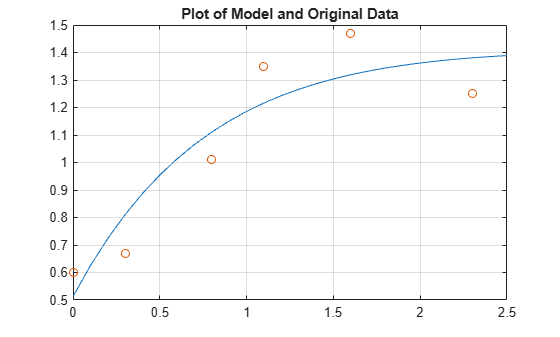### Multiple Regression

This example shows how to use multiple regression to model data that is a function of more than one predictor variable.

When y is a function of more than one predictor variable, the matrix equations that express the relationships among the variables must be expanded to accommodate the additional data. This is called multiple regression.

Measure a quantity $y$ for several values of ${x}_{1}$ and ${x}_{2}$. Store these values in vectors `x1`, `x2`, and `y`, respectively.

```x1 = [.2 .5 .6 .8 1.0 1.1]'; x2 = [.1 .3 .4 .9 1.1 1.4]'; y = [.17 .26 .28 .23 .27 .24]';```

A model of this data is of the form

`$y={a}_{0}+{a}_{1}{x}_{1}+{a}_{2}{x}_{2}.$`

Multiple regression solves for unknown coefficients ${a}_{0}$, ${a}_{1}$, and ${a}_{2}$ by minimizing the sum of the squares of the deviations of the data from the model (least-squares fit).

Construct and solve the set of simultaneous equations by forming a design matrix, `X`.

`X = [ones(size(x1)) x1 x2];`

Solve for the parameters by using the backslash operator.

`a = X\y`
```a = 3×1 0.1018 0.4844 -0.2847 ```

The least-squares fit model of the data is

`$y=0.1018+0.4844{x}_{1}-0.2847{x}_{2}.$`

To validate the model, find the maximum of the absolute value of the deviation of the data from the model.

```Y = X*a; MaxErr = max(abs(Y - y))```
```MaxErr = 0.0038 ```

This value is much smaller than any of the data values, indicating that this model accurately follows the data.

### Programmatic Fitting

This example shows how to use MATLAB functions to:

Load sample census data from `census.mat`, which contains U.S. population data from the years 1790 to 1990.

`load census`

This adds the following two variables to the MATLAB workspace.

• `cdate` is a column vector containing the years 1790 to 1990 in increments of 10.

• `pop` is a column vector with the U.S. population numbers corresponding to each year in `cdate`.

Plot the data.

```plot(cdate,pop,'ro') title('U.S. Population from 1790 to 1990')```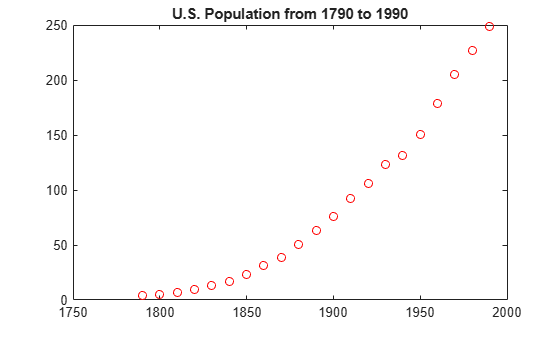The plot shows a strong pattern, which indicates a high correlation between the variables.

#### Calculate Correlation Coefficients

In this portion of the example, you determine the statistical correlation between the variables `cdate` and `pop` to justify modeling the data. For more information about correlation coefficients, see Linear Correlation.

Calculate the correlation-coefficient matrix.

`corrcoef(cdate,pop)`
```ans = 2×2 1.0000 0.9597 0.9597 1.0000 ```

The diagonal matrix elements represent the perfect correlation of each variable with itself and are equal to 1. The off-diagonal elements are very close to 1, indicating that there is a strong statistical correlation between the variables `cdate` and `pop`.

#### Fit a Polynomial to the Data

This portion of the example applies the `polyfit` and `polyval` MATLAB functions to model the data.

Calculate fit parameters.

`[p,ErrorEst] = polyfit(cdate,pop,2);`

Evaluate the fit.

`pop_fit = polyval(p,cdate,ErrorEst);`

Plot the data and the fit.

```plot(cdate,pop_fit,'-',cdate,pop,'+'); title('U.S. Population from 1790 to 1990') legend('Polynomial Model','Data','Location','NorthWest'); xlabel('Census Year'); ylabel('Population (millions)');```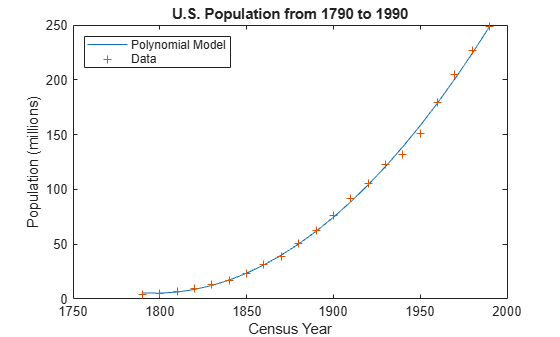The plot shows that the quadratic-polynomial fit provides a good approximation to the data.

Calculate the residuals for this fit.

```res = pop - pop_fit; figure, plot(cdate,res,'+') title('Residuals for the Quadratic Polynomial Model')```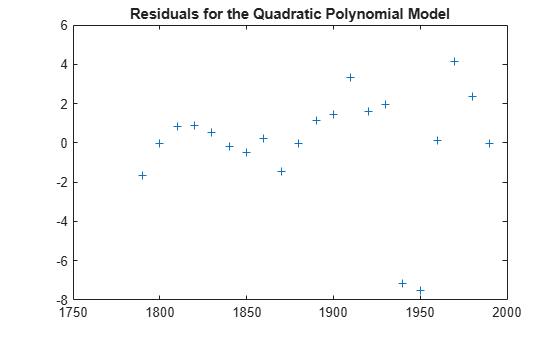Notice that the plot of the residuals exhibits a pattern, which indicates that a second-degree polynomial might not be appropriate for modeling this data.

#### Plot and Calculate Confidence Bounds

Confidence bounds are confidence intervals for a predicted response. The width of the interval indicates the degree of certainty of the fit.

This portion of the example applies `polyfit` and `polyval` to the `census` sample data to produce confidence bounds for a second-order polynomial model.

The following code uses an interval of $±2\Delta$, which corresponds to a 95% confidence interval for large samples.

Evaluate the fit and the prediction error estimate (delta).

`[pop_fit,delta] = polyval(p,cdate,ErrorEst);`

Plot the data, the fit, and the confidence bounds.

```plot(cdate,pop,'+',... cdate,pop_fit,'g-',... cdate,pop_fit+2*delta,'r:',... cdate,pop_fit-2*delta,'r:'); xlabel('Census Year'); ylabel('Population (millions)'); title('Quadratic Polynomial Fit with Confidence Bounds') grid on```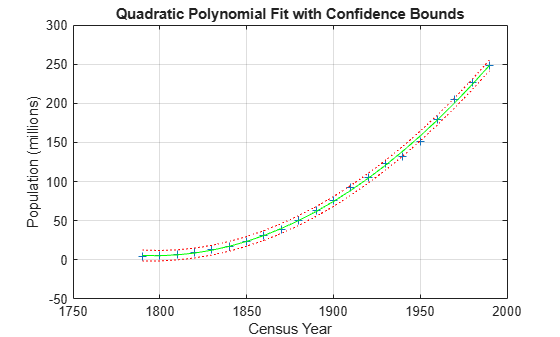The 95% interval indicates that you have a 95% chance that a new observation will fall within the bounds.